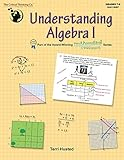# Understanding Algebra IUnderstanding Algebra I is part of the Mathematical Reasoning series for elementary grades which is among my Top Picks. It can be used as a high school level algebra course, but it should also be excellent for junior high students who are ready to tackle algebra.

It is available in print or as an ebook. While it is not as colorful or as heavily illustrated as the books for the younger grade levels, it has more color and illustrations than most first-year algebra texts. It has 318 pages of text plus a 36-page answer key. A free solutions PDF is available from the publisher.

It seems to be a more user-friendly course than some others. Explanations are thorough, sometimes including illustrations, graphics, and charts. A few lessons in the unit on polynomials even show how tiles (or Base Ten Blocks or similar manipulatives that include squares that can represent x 2) can be used to demonstrate multiplication of binomials. Lessons are written directly to the student, so no teaching is required.

Lessons cover the same essential content as most algebra 1 courses: sets, operations, properties, evaluating expressions, solving equations, applying algebra in words problems, inequalities, polynomials, factoring, radicals, various types of functions, quadratic equations, the quadratic formula, and various ways of solving systems of equations and inequalities.

Some lessons teach calculator usage with step-by-step directions, but they point out when calculators are useful and do not force students to rely only on the calculator.

Just as in the other Mathematical Reasoning courses, there are many word problems (e.g., time/rate/distance problems, consecutive integer problems, coin problems, and percent and ratio problems) with extensive teaching on strategies to use plus plenty of practical application and critical thinking activities. Many exercises challenge students to find and explain errors in problem solving while others ask students to explain their thinking. The chapters on word problems are particularly useful for test preparation since they present the types of word problems commonly encountered on standardized tests.

While I very much like Understanding Algebra I for its style of presentation, it lacks two important features: cumulative review and tests. Chapter questions only address topics taught in each chapter. You can try to fill these gaps with free online resources. I found a few with a quick search, but there are certainly many other options. Mathopolis has free practice problems but only for selected topics while math.com has a free worksheet generator (with answer keys) that will present various types of problems that you can select. Varsity Tutors offers free online practice tests that are scored by the computer. While none of these correlates exactly with Understanding Algebra I, they (or similar sites) might provide cumulative review and tests sufficient for your purposes.

### Pricing Information

When comparison prices appear, please keep in mind that they are subject to change. Click on links where available to verify price accuracy.

List Price for either print or ebook: \$39.99

#### Understanding Algebra IGet a FREE subscription to Cathy's E-Newsletter

### My Lists

Save reviews to lists to guide curriculum choices. Register or log in to start your first list.

### Instant Key

• Learning Environment: independent study